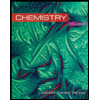# Estimate the percentage of the total mass of a 58Ni atom that is due to (a) electrons, (b) protons, and (c) neutrons by assuming that the mass of the atom is simply the sum of the masses of the appropriate numbers of subatomic particles. The mass of an electron is 0.00054858 amu, the mass of a proton is 1.0073 amu, and the mass of a neutron is 1.0087 amu. a. % by mass e- b, % by mass p c.90 by mass n- 0 0 0 0

Question
Expert Solution

### Want to see the full answer?

Check out a sample Q&A hereStudents who’ve seen this question also like:Chemistry
10th Edition
ISBN: 9781305957404
Author: Steven S. Zumdahl, Susan A. Zumdahl, Donald J. DeCoste
Publisher: Cengage Learning